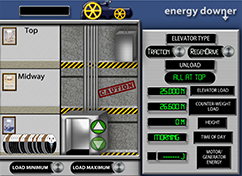# Energy DownerElevators travel up and down all day long in a busy office building. Sometimes the elevator has a large load, while other times only a few people are on-board. Very often useful energy can be extracted when objects move from a higher position to a lower one. Do you think useful energy can be recovered from a moving elevator?

### What is a load and how is it measured?

In an elevator system, the load is the total weight of the elevator and passengers that must be lifted up from one height to another. Weight is the force due to gravity. Since weight is a force, weight is measured in force units. The English weight unit is pounds, abbreviated as "lbs." The metric unit of weight is the newton, abbreviated as "N".

### What is the typical unit for measuring energy?

Energy is defined as the ability to do work. The physics definition of work is force times the distance an object is moved by the force. The metric unit of energy is called the joule, abbreviated as "J." One joule is defined as 1 newton times 1 meter. In an elevator, energy is required to move the elevator load a given distance in the vertical direction.

### What is potential energy

Potential energy is stored energy. There are various forms of potential energy, including elastic, chemical, electrical, and gravitational. An elevator must do work against gravity in lifting the load from the ground floor to higher floors. The energy associated with this lifting process can be calculated using the gravitational potential energy (GPE) equation, which states that: GPE = mgh, where m = mass in grams or kilograms, g = gravitational acceleration, which is 9.8 meters/second squared, and h = height lifted in meters.

### What is kinetic energy

Kinetic energy is energy due to the motion of an object. Any moving object has kinetic energy and can do work on another object. The larger the mass of an object that's in motion, the greater the kinetic energy of that moving object. The faster the mass is moving, the greater the amount of kinetic energy associated with that moving object.

### What is the Law of Conservation of Energy?

The Law of Conservation of Energy states that the total energy in the universe is constant. Energy can be transferred from one object to another or transformed into different types of energy, but the total energy may never be increased or decreased. The general equation that demonstrates this law is: the total energy = the sum of the potential energy and kinetic energy of a system. Any gains in the potential energy of a system must be counteracted with a loss in kinetic energy of that system.

### What is waste thermal energy?

In any process that involves motion, some of the energy associated with that process is converted into another form of energy, mainly heat. For instance, a pendulum set in motion eventually stops because with each back and forth swing, some of the kinetic energy of the pendulum is reduced due to the friction between the supporting string and its point of attachment. The friction changes some of the kinetic energy into heat, that is given off to the environment. This removal of energy from the pendulum system eventually causes the pendulum to slow down and stop swinging entirely.

### How does a typical traction elevator work?

Most elevators are traction elevators. This type of elevator uses an electrical motor to raise and lower passengers from one floor to another in a building. These elevator systems typically consist of an elevator passenger car connected via a strong cable strung over a pulley to a heavy counterweight. The counterweight is used to balance the load in the elevator car and reduce the amount of energy required to raise and lower the elevator. As the elevator car ascends, the counterweight descends. Large amounts of energy are expended by the system when full elevator loads are raised and empty loads are lowered. In all cases, traction elevator systems consume electrical energy, which is converted to mechanical motion (lifting or lowering) and waste heat.

### How does a regenerative drive elevator work?

A regenerative drive elevator, called a Regen elevator, differs from a typical traction elevator in the system's ability to produce electrical energy given certain conditions. Traction elevators always only consume electrical energy. Regen systems convert gravitational potential energy (GPE) to electrical energy via a generator that is part of the elevator system. Anytime the load or counterweight that is moving downward has a greater weight than the load or counterweight moving upward, a portion of the GPE of the larger falling weight is converted to electricity using the system's generator.